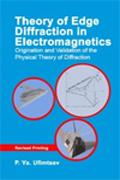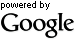# Theory of Edge Diffraction in Electromagnetics• Book title: origination and validation of the physical theory of diffraction, Revised

• Author:

• Year: 2000

• Format: Hardback

• Product Code: SBEW0540

• ISBN: 978-1-89112-166-1

• Pagination: 444pp

• Stock Status: Out of stock

The estimated arrival date is June 2012

## Description

This book is an essential resource for researchers involved in designing antennas and RCS calculations. It is also useful for students studying high frequency diffraction techniques. It contains basic original ideas of the Physical Theory of Diffraction (PTD), examples of its practical application, and its validation by the mathematical theory of diffraction. The derived analytic expressions are convenient for numerical calculations and clearly illustrate the physical structure of the scattered field.

Key Features

Theory of diffraction at black bodies introduces the Shadow Radiation, a fundamental component of the scattered field.

RCS of finite bodies of revolution-cones, paraboloids, etc. -models of construction elements for aircraft and missiles.

Scheme for measurement of that part of a scattered field which is radiated by the diffraction (so-called nonuniform) currents induced on scattering objects.

Development of the parabolic equation method for investigation of edge-diffraction.

New exact and asymptotic solutions in the strip diffraction problems, including scattering at an open resonator.

## Book contents

1. Diffraction of Electromagnetic Waves at Black Bodies: Generalization of Kirchhoff-Kottler Theory

2. Edge Diffraction at Convex Perfectly Conducting Bodies: Elements of the Physical Theory of Diffraction

3. Edge Diffraction at Concave Surfaces: Extension of the Physical Theory of Diffraction

4. Measurement of Radiation from Diffraction / Nonuniform Currents

5. Analysis of Wedge Diffraction Using the Parabolic Equation Method

6. Current Waves on Thin Conductors and Strips

7. Radiation of Edge Waves: Theory Based on the Reciprocity Theorem

8. Functional and Integral Equations for Strip Diffraction (Neumann Boundary Problem)

9. Asymptotic Representation for the Current Density on a Strip

10. Asymptotic Representation for the Scattering Pattern

11. Plane Wave Diffraction at a Strip Oriented in the Direction of Polarization (Dirichlet Boundary Problem)

12. Edge Diffraction at Open-Ended Parallel Plate Resonator

Conclusions

References

Appendix: Relationships Between the Gaussian System (GS) and the System International (SI) for Electromagnetic Units

IndexSearch the full text of this book